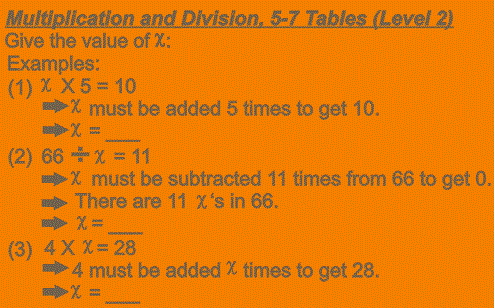# Multiplication And Division Tables: 5-7 Tables (Level 2)

216 QuestionsMultiplication and Division tables – 5 to 7 Tables (Level 2). This quiz contains 216 questions. There is an ϰ involved in every question of which the correct value must be given in the answer. Examples: (1) 2 X ϰ = 10 ⇒ 2 must be added ϰ times to get 10 ⇒ ϰ = ___ (2) ϰ ÷ 7 = 5 ⇒ 7 must be subtracted 5 times from ϰ to get 0 ⇒ There are 5 7’s in x ⇒ ϰ = ___ The general question for this quiz is: Give the correct value of ϰ. This quiz will improve your knowledge of the 5-7 Multiplication and Division Tables.